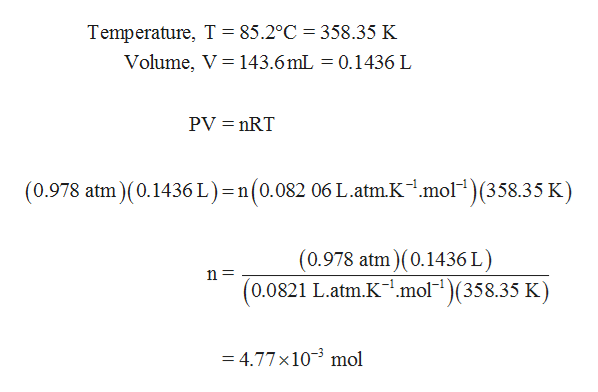# Consider 0.338 g of a volatile liquid that as a gas occupies 143.6 mL at a pressure of 0.978 atm at 85.2 C. What is the mass of the volatile liquid?

Question
10 views

Consider 0.338 g of a volatile liquid that as a gas occupies 143.6 mL at a pressure of 0.978 atm at 85.2 C. What is the mass of the volatile liquid?

check_circle

Step 1

Note:

Thank you for question. Since the mass of the volatile liquid is already given, we are determining the molar mass of the volatile liquid.

Step 2

Number of moles of volatile liquid can be calcul...help_outlineImage TranscriptioncloseTemperature, T = 85.2°C = 358.35 K Volume, V 143.6 mL = 0.1436 L PV nRT (0.978 atm)(0.1436 L) = n(0.082 06 L.atm.K1.mol(358.35 K) (0.978 atm) (0.1436 L) (0.0821 L.atm.K.mol )(358.35 K) n= = 4.77 x103 mol fullscreen

### Want to see the full answer?

See Solution

#### Want to see this answer and more?

Solutions are written by subject experts who are available 24/7. Questions are typically answered within 1 hour.*

See Solution
*Response times may vary by subject and question.
Tagged in
ScienceChemistry

### General Chemistry